#### Problem (1)

Given the following information:Compute the curve components, endpoint stations, and radial deflections to full stations.

Curve components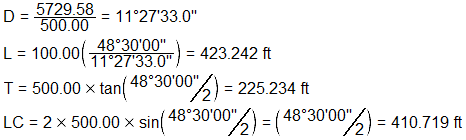Endpoint stationing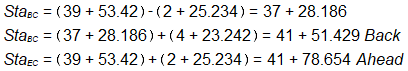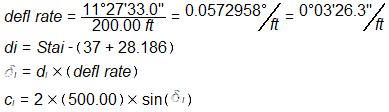Curve table

 Station di, ft δi ci, ft EC Back 41+51.429 423.242 = L 24°15'00.0" = Δ/2 410.719 = LC 41+00 371.814 21°18'12.1" 363.306 40+00 271.814 15°34'25.6" 268.479 39+00 171.814 9°50'39.1" 170.970 38+00 71.814 4°06'52.6" 71.752 BC 37+28.186 0.000 0°00'00" 0.000

Deflection angles are all to the right.

#### Problem (2)

For the conditions below:What are the deflection angle and radial chord at the BC to curve station 41+00.00?

Compute ΔDetermine BC station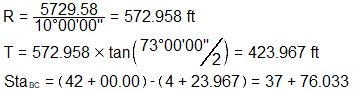Set up and solve deflection equations for station 41+00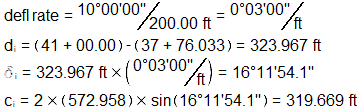Answer: 319.67 ft at 16°11'54" Left.